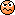# Indexing formulas

## Main Question or Discussion Point

How to number formulas and how to refer to formulas in PhysicsForums?

Related Feedback and Announcements News on Phys.org
mfb
Mentor
You can give them numbers manually, see formula 1. You can't add links, but if the post is so long that links are needed then the forum is probably not the right place for it anyway.
$$\frac{\pi^2}{6}=\sum_{n=1}^\infty \frac{1}{n^2}\quad\quad (1)$$

•Greg Bernhardt

Ibix
You can put equations in the eqnarray environment. $$\begin{eqnarray} F&=&ma \end{eqnarray}$$But as far as I know, if someone quotes me the quote will have equation 2. So it's not great...

Ibix
You can put equations in the eqnarray environment. $$\begin{eqnarray} F&=&ma \end{eqnarray}$$But as far as I know, if someone quotes me the quote will have equation 2. So it's not great...
Let's test that claim. Edit: yep.

BvU
Homework Helper
2019 Award
You can even let $\LaTeX#3 do the numbering: with the line \begin{equation} a=b \\ c = d \end {equation} twice I get: \begin{equation} a=b \\ c = d \end {equation} \begin{equation} a=b \\ c = d \end {equation} However, MathJax or latex itself does the numbering per page, so after a few previews, post #4 had the number$(9)$and post #5 had the number$(9)$\begin{equation} a=b \\ c = d \\ e=f \end {equation} \begin{equation} a=b \\ c = d \\ e=f \end {equation} and twice previewing thispost, this one is at$(19)$whereas you might see$(13)$!? [edit:] nope,$\it 6! Ibix Science Advisor ...and @BvU's counter continues from mine plus my own quotation of mine. So if you type a response to either of us and, while you're typing, someone else replies, your numbers will be affected and you'll need to adjust your references. I'd recommend against using the capability - even in the OP, since quotations from it will have different numbers.BvU Science Advisor Homework Helper 2019 Award Same numbering problem with \begin {align} @mfb : move toLaTeX$forum ? And perhaps ask the Latexhelp author (who is it ?) to expand on the problem in 'multiple lines' where the \begin { } ... \end{...} environment is mentioned. Wasn't there a way to restart the automatic numbering ? seems that \setcounter{equation}{1} is$\mbox{AMS}\TeX$($\AMSTeX $?), not$\LaTeX##.

In the mean time I found here that \def is admissible, but I didn't find a way to load a set of \def comfortably ... (Phrases I use often but only once per post)
and it doesn't like BB code - understandably

(thinking of \def\PFg{PF guidelines})​

jim mcnamara
Mentor
I think @fresh_42 uses some key macros and may have a way of preloading \def as well. Let's see what he says.

fresh_42
Mentor
I think @fresh_42 uses some key macros and may have a way of preloading \def as well. Let's see what he says.
I use a script (AutoHotkey) which allows me to define my own shortcuts, so I can put long words on a single key. In cases like
Phrases I use often
e.g. in order to type [Moderator's note: Moved from a technical forum and thus no template.] I hit Ctrl+>.

•jim mcnamara